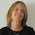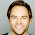Monday, November 17, 2014

High School Math Solutions – Polynomial Long Division Calculator

Polynomial long division is very similar to numerical long division where you first divide the large part of the number, multiply the answer by the divisor, subtract and continue dividing the reminder until the reminder is smaller than the divisor.

With polynomials you do the same, only now you have to divide the higher order terms (first term of the numerator divide by the first term of the denominator).

Cheers,
Michal

1.1.2.3.4.